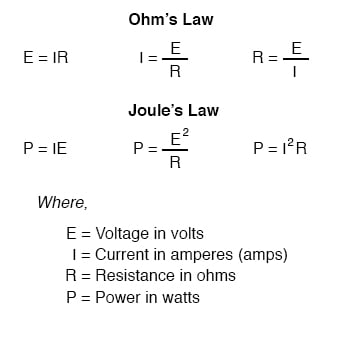# DC Circuit Equations and Laws

## Chapter 1 - Useful Equations And Conversion Factors

### Power Equation of Ohm’s Law and Joule’s LawNOTE: the symbol “V” (“U” in Europe) is sometimes used to represent voltage instead of “E”.

In some cases, an author or circuit designer may choose to exclusively use “V” for voltage, never using the symbol “E.”

Other times the two symbols are used interchangeably, or “E” is used to represent voltage from a power source while “V” is used to represent voltage across a load (voltage “drop”).

### Kirchhoff’s Voltage and Current Laws

“The algebraic sum of all voltages in a loop must equal zero.”
Kirchhoff’s Voltage Law (KVL)
“The algebraic sum of all currents entering and exiting a node must equal zero.”
Kirchhoff’s Current Law (KCL)

RELATED WORKSHEETS:

• Share
Published under the terms and conditions of the Design Science License